# What are various ladder logic symbols?

Traditional relay logic control circuits were utilised to develop the ladder logic symbols used in PLC programming. If you have a rudimentary understanding of electric circuits, you should have no trouble getting started with ladder logic programming. If not, don’t panic; ladder logic is a graphical programming language, thus learning the fundamental ladder logic symbols and principles is simple.

The basic programming components used in ladder diagrams are ladder logic symbols. Ladder logic symbols can be used separately or in combination to construct logic instructions in PLC programming. Originally designed for bit logic operations, ladder logic symbols today incorporate higher-level functions like timers, counters, math, comparison, PID loops, data manipulation, and data translation.

Normally Open (NO)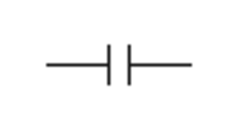Its operation is quite simple. When the condition is met, the contact is closed and the output logic flow is activated. When the condition is FALSE, the contact is OPEN, and the output logic flow is interrupted.

How does the Normally Open Contact Work?

Initially, the contact was connected to a relay coil. The contact would close when the relay’s coil was energised. The ladder logic symbol works similarly. It specifies a logical bit that can be set to 0 (LOW) or 1 (HIGH) (HIGH). The instruction will evaluate to TRUE or FALSE depending on the state. If the instruction is TRUE, the current will be allowed to flow and the PLC will be able to assess the next instruction. If it is FALSE, the ladder logic symbol will terminate the execution.

Common Uses Of Normally Open Symbol:

• Start Push buttons.
• Selector switches.
• Digital instrumentation.
• Internal programming.

Normally Closed Contact (NC) Symbol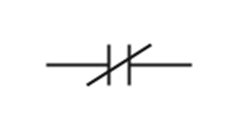When the condition is met, the contact is OPEN, and the output logic flow is obstructed. When the condition is FALSE, the contact is CLOSED and the output logic flow is activated. The operation of the NC contact symbol is the inverse of that of the NO contact symbol.

How does the Normally Closed Contact Work?

The normally closed contact would also be connected to a solid-state relay’s coil. When there is no current flowing through the coil, the contact allows current to flow through. When the coil is activated, however, no current flows through the contact. In PLC programming ladder logic, the NC Contact or XIO instruction would function similarly. In other words, when the bit is LOW, current can flow through, but when it is HIGH, no current can flow through.

Common uses Of Normally Closed Symbol

• Stop Push buttons.
• Fail safe instrumentation.
• Internal programming.

Output Coil Symbol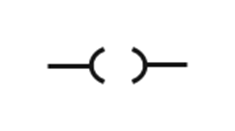If the input condition is TRUE, the output condition is ON. The output is OFF if the input condition is FALSE.

Common uses of Output Coil Symbol

• Motor control.
• Actuator control.
• Indication lamps.
• Warning sirens.
• Internal programming.
• Sequence logic.

One Shot Symbol– Positive Edge Detection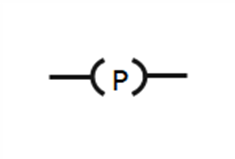When the input condition changes from FALSE to TRUE, the output is ON for the duration of one PLC scan.

Common uses of One Shot Symbol:

• Counting applications.
• Math commands.
• Data Transfer commands.
• Latch output during a specific set of conditions.

Set Coil Symbol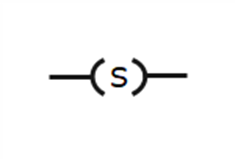When the input condition changes from false to true, the output is turned ON, and once set ON, it remains ON even if the input condition changes to FALSE.

Reset Coil Symbol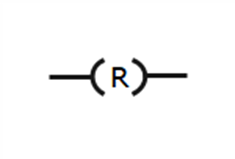When the input condition is met, the output is turned off. When the input condition is false, the output has no effect. The SET and RESET coils can share the same variable address and hence function together.

Timer Delay on Symbol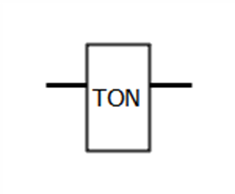The timer starts when the input condition is true. When the current time set point is reached, the output turns ON. If the input condition is FALSE at any point, the timer ends and the output is turned off.

Timer Delay-off Symbol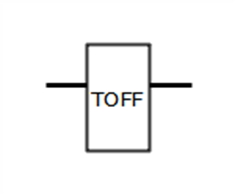When the input condition is met, the output is activated. If the input condition is false, the timer starts, and when the preset time set point is reached, the output is turned off. If the input condition becomes TRUE at any point, the timer is stopped and the output is turned ON.

PID ControllerThe PID Controller is used to regulate the process value. The principal element (Input) is used to measure the process variable, and the output is adjusted to keep the process variable value at the set point input value. The Proportional, Integral, and Derivative input values are tuned to improve control performance. This is done in accordance with the process criteria.

Counter Up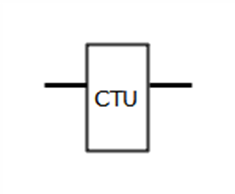When the input changes from False to True, the counter increases by one value from the last stored value. When this stored value reaches the preset value, the output changes to True, and the stored value is reset to 0.

Counter Down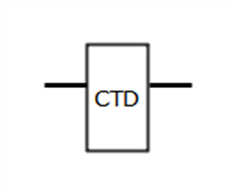When the input changes from True to False, the counter lowers by one value from the latest stored value. When this stored value hits zero, the output changes to True and the stored value is reset to the preset value.

1 Like